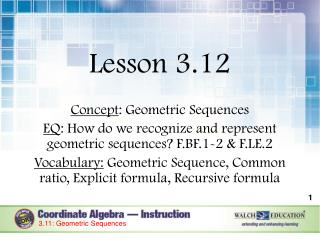DownloadDownload PresentationLesson 3.12 Concept : Geometric Sequences

Lesson 3.12 Concept : Geometric Sequences

Télécharger la présentationLesson 3.12 Concept : Geometric Sequences

- - - - - - - - - - - - - - - - - - - - - - - - - - - E N D - - - - - - - - - - - - - - - - - - - - - - - - - - -
Presentation Transcript

1. Lesson 3.12 Concept: Geometric Sequences EQ: How do we recognize and represent geometric sequences? F.BF.1-2 & F.LE.2 Vocabulary: Geometric Sequence, Common ratio, Explicit formula, Recursive formula 3.11: Geometric Sequences

2. Activator: First Word Using the word ‘EXPONENTIAL’, create a phrase starting with each letter in the word on a sheet of paper. To get you started, I will give you an example. Exponential graphs looks like a ‘J’ curve.XPONENTIAL Now you finish the rest. 3.8.2: Geometric Sequences

3. Introduction A geometric sequence is a list of terms separated by a common ratio, r, which is the number multiplied by each consecutive term in a geometric sequence. A geometric sequence is an exponential function with a domain of whole numbers in which the ratio between any two consecutive terms is equal. 3.11: Geometric Sequences

4. Introduction (continued) Just like arithmetic sequences, Geometric sequencescan be represented by formulas, either explicit or recursive, and those formulas can be used to find a certain term of the sequence or the number of a certain value in the sequence. Recall A recursive formula is a formula used to find the next term of a sequence when the previous term is known. An explicit formula is a formula used to find the nth term of a sequence. 3.11: Geometric Sequences

5. Formulas and their Purpose Geometric Sequences Explicit Formula: “Finds a specific term” Recursive Formula: r “Uses previous terms to find the next terms” First Term Current Term Common Ratio Previous Term 3.11: Geometric Sequences

6. Steps to create formulas and solve for geometric sequences Find the common ratio bydividing the 2nd term by the 1stterm. Decide which formula to use. (explicit or recursive) Substitute your values to create your formula. Find the specific term if asked to do so. 3.8.2: Geometric Sequences

7. Guided Practice Example 1 Create the recursive formula that defines the sequence: A geometric sequence is defined by 2, 8, 32, 128, … 3.11: Geometric Sequences

8. Guided Practice Example 1, continued Create the recursive formula that defines the sequence: A geometric sequence is defined by 2, 8, 32, 128, … 3.11: Geometric Sequences

9. Guided Practice Example 2 Create the recursive formula that defines the sequence: A geometric sequence is defined by 45, -15, 5, , … 3.11: Geometric Sequences

10. Guided Practice Example 2, continued Create the recursive formula that defines the sequence: A geometric sequence is defined by 45, -15, 5, , … 3.11: Geometric Sequences

11. You Try 1 Use the following sequence to create a recursive formula. 10, -30, 90, -270, … 3.11: Geometric Sequences

12. Guided Practice Example 3 A geometric sequence is defined recursively by an= an – 1·, with a1 = 6. Find the first 5 terms of the sequence. Using the recursive formula: a1 = 6 a2 = a1· a2 = a3 = a4 = a5= The first five terms of the sequence are: 3.11: Geometric Sequences

13. Guided Practice – Example 4 A geometric sequence is defined recursively by an= an – 1·, with a1 = 3000. Find the first 5 terms of the sequence. Using the recursive formula: a1 = 3000 a2 = a1· a2 = 3000 ·= 300 a3 = 300 · = 30 a4 = 30 · = 3 a5 = 3 · = The first five terms of the sequence are: 3000, 300, 30, 3, and . 3.11: Geometric Sequences

14. You Try 2 An arithmetic sequence is defined recursively by · 6, with a1= 0.2 Find the first 5 terms of the sequence. 3.11: Geometric Sequences

15. Guided Practice Example 5 Write an explicit formula to represent the sequence from example 1, and find the 10th term. The first five terms of the sequence are: 2, 8, 32, 128, and 512. 3.11: Geometric Sequences

16. Guided Practice: Example 5, continued The first five terms of the sequence are: 2, 8, 32, 128, and 512. 3.11: Geometric Sequences

17. Guided Practice Example 6 Write an explicit formula to represent the sequence from example 3, and find the 15th term. The first five terms of the sequence are: 6, -18, 54, -162, and 486 3.11: Geometric Sequences

18. Guided Practice: Example 6, continued The first five terms of the sequence are: 6, -18, 54, -162, and 486 3.11: Geometric Sequences

19. You Try 3 Use the following sequence to create an explicit formula. Then find . 4, 8, -16, 32, … 3.11: Geometric Sequences

20. Summary: Last word Using the word ‘GEOMETRIC’, create a phrase with each letter just like with exponential from before. 3.8.2: Geometric Sequences